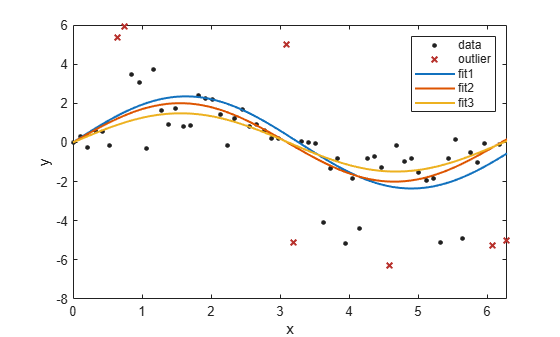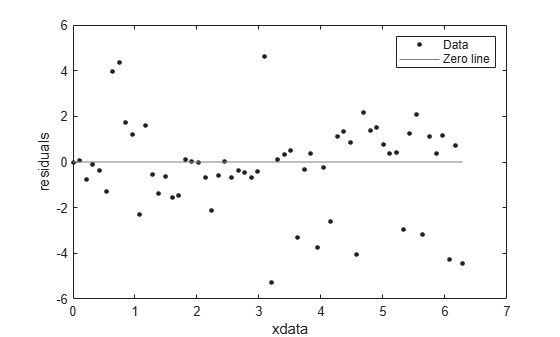Main Content

# plot

Plot `cfit` or `sfit` object

## Syntax

``plot(sfit)``
``plot(sfit, [x, y], z)``
``H = plot(sfit, ..., Name,Value)``
``H = plot(sfit, ...)``
``plot(cfit)``
``plot(cfit,x,y)``
``plot(cfit,x,y,DataLineSpec)``
``plot(cfit,FitLineSpec,x,y,DataLineSpec)``
``plot(cfit,x,y,outliers)``
``plot(cfit,x,y,outliers,OutlierLineSpec)``
``plot(...,ptype,...)``
``plot(...,ptype,level)``
``H = plot(...)``

## Description

``` NoteThese syntaxes are available for surfaces, or `sfit` objects. `plot(sfit)` plots the `sfit` object over the range of the current axes, if any, or otherwise over the range stored in the fit.```
````plot(sfit, [x, y], z)` plots z versus x and y and plots `sfit` over the range of x and y.```
````H = plot(sfit, ..., Name,Value)` selects which way to plot the surface fit object `sfit`.```
````H = plot(sfit, ...)` returns a vector of handles of the plotted objects.```

example

``` NoteThese syntaxes are available for curves, or `cfit` objects. `plot(cfit)` plots the `cfit` object over the domain of the current axes, if any. If there are no current axes, and `fun` is an output from the `fit` function, the plot is over the domain of the fitted data.```
````plot(cfit,x,y)` plots `cfit` together with the predictor data `x` and the response data `y`.```
````plot(cfit,x,y,DataLineSpec)` plots the predictor and response data using the color, marker symbol, and line style specified by the `DataLineSpec` formatting character vector.```
````plot(cfit,FitLineSpec,x,y,DataLineSpec)` plots `fun` using the color, marker symbol, and line style specified by the `FitLineSpec` formatting character vector, and plots `x` and `y` using the color, marker symbol, and line style specified by the `DataLineSpec` formatting character vector.```
````plot(cfit,x,y,outliers)` plots data indicated by `outliers` in a different color. `outliers` can be an expression describing a logical vector, e.g., `x > 10`, a vector of integers indexing the points you want to exclude, e.g., `[1 10 25]`, or a logical array where `true` represents an outlier. You can create the array with `excludedata`.```
````plot(cfit,x,y,outliers,OutlierLineSpec)` plots `outliers` using the color, marker symbol, and line style specified by the `OutlierLineSpec`.```
````plot(...,ptype,...)` uses the plot type specified by `ptype`.```
````plot(...,ptype,level)` plots prediction intervals with a confidence level specified by `level`. ```
``` NoteThis syntax is available for both curves and surfaces. Plot types can be single or multiple, with multiple plot types specified as a cell array of character vectors. With a single plot type, `plot` draws to the current axes and can be used with commands like `hold` and `subplot`. With multiple plot types, `plot` creates subplots for each plot type. `H = plot(...)` returns a vector of handles to the plotted objects.```

## Examples

collapse all

This example shows how to plot the data, the outliers, and the results of three fit objects with different colors and line styles.

Create a baseline sinusoidal signal.

```xdata = (0:0.1:2*pi)'; y0 = sin(xdata);```

Add noise to the signal with non-constant variance.

```% Response-dependent Gaussian noise gnoise = y0.*randn(size(y0)); % Salt-and-pepper noise spnoise = zeros(size(y0)); p = randperm(length(y0)); sppoints = p(1:round(length(p)/5)); spnoise(sppoints) = 5*sign(y0(sppoints)); ydata = y0 + gnoise + spnoise;```

Fit the noisy data with a baseline sinusoidal model.

```f = fittype('a*sin(b*x)'); fit1 = fit(xdata,ydata,f,'StartPoint',[1 1]);```

Identify “outliers” as points at a distance greater than 1.5 standard deviations from the baseline model, and refit the data with the outliers excluded.

```fdata = feval(fit1,xdata); I = abs(fdata - ydata) > 1.5*std(ydata); outliers = excludedata(xdata,ydata,'indices',I); fit2 = fit(xdata,ydata,f,'StartPoint',[1 1],... 'Exclude',outliers);```

Compare the effect of excluding the outliers with the effect of giving them lower bisquare weight in a robust fit.

`fit3 = fit(xdata,ydata,f,'StartPoint',[1 1],'Robust','on');`

Plot the data, the outliers, and the results of the fits.

```plot(fit1,'r-',xdata,ydata,'k.',outliers,'m*') hold on plot(fit2,'c--') plot(fit3,'b:') xlim([0 2*pi])```Plot the residuals for the two fits considering outliers.

```figure plot(fit2,xdata,ydata,'co','residuals') hold on plot(fit3,xdata,ydata,'bx','residuals') hold off```Load data and fit a Gaussian, excluding some data with an expression, then plot the fit, data and the excluded points.

```[x, y] = titanium; f1 = fit(x',y','gauss2', 'Exclude', x<800); plot(f1,x,y,x<800)```## Input Arguments

collapse all

Fit object to plot, specified as an `sfit` object.

Data to plot, specified as a matrix with either one (curve fitting) or two (surface fitting) columns.

Data to plot, specified as a matrix with either one (curve fitting) or two (surface fitting) columns.

Data to plot, specified as a matrix with either one (curve fitting) or two (surface fitting) columns.

Fit object to plot, specified as a `cfit` object.

• An expression describing a logical vector, e.g., `x > 10`.

• A vector of integers indexing the points you want to exclude, e.g., `[1 10 25]`.

• A logical vector for all data points where `true` represents an outlier, created by `excludedata`.

For an example, see Exclude Points from Fit.

Data Types: `logical` | `double`

Line style, marker, and color used to plot the predictor data x and response data y, specified as a character vector or string containing symbols. The symbols can appear in any order. You do not need to specify all three characteristics (line style, marker, and color). For example, if you omit the line style and specify the marker, then the plot shows only the marker and no line.

Example: `'--or'` is a red dashed line with circle markers

Line StyleDescription
`-`Solid line (default)
`--`Dashed line
`:`Dotted line
`-.`Dash-dot line
MarkerDescription
`o`Circle
`+`Plus sign
`*`Asterisk
`.`Point
`x`Cross
`s`Square
`d`Diamond
`^`Upward-pointing triangle
`v`Downward-pointing triangle
`>`Right-pointing triangle
`<`Left-pointing triangle
`p`Pentagram
`h`Hexagram
ColorDescription

`y`

yellow

`m`

magenta

`c`

cyan

`r`

red

`g`

green

`b`

blue

`w`

white

`k`

black

Line style, marker, and color used to plot the cfit function, specified as a character vector or string containing symbols. The symbols can appear in any order. You do not need to specify all three characteristics (line style, marker, and color). For example, if you omit the line style and specify the marker, then the plot shows only the marker and no line.

Example: `'--or'` is a red dashed line with circle markers

Line StyleDescription
`-`Solid line (default)
`--`Dashed line
`:`Dotted line
`-.`Dash-dot line
MarkerDescription
`o`Circle
`+`Plus sign
`*`Asterisk
`.`Point
`x`Cross
`s`Square
`d`Diamond
`^`Upward-pointing triangle
`v`Downward-pointing triangle
`>`Right-pointing triangle
`<`Left-pointing triangle
`p`Pentagram
`h`Hexagram
ColorDescription

`y`

yellow

`m`

magenta

`c`

cyan

`r`

red

`g`

green

`b`

blue

`w`

white

`k`

black

Line style, marker, and color used to plot the outliers, specified as a character vector or string containing symbols. The symbols can appear in any order. You do not need to specify all three characteristics (line style, marker, and color). For example, if you omit the line style and specify the marker, then the plot shows only the marker and no line.

Example: `'--or'` is a red dashed line with circle markers

Line StyleDescription
`-`Solid line (default)
`--`Dashed line
`:`Dotted line
`-.`Dash-dot line
MarkerDescription
`o`Circle
`+`Plus sign
`*`Asterisk
`.`Point
`x`Cross
`s`Square
`d`Diamond
`^`Upward-pointing triangle
`v`Downward-pointing triangle
`>`Right-pointing triangle
`<`Left-pointing triangle
`p`Pentagram
`h`Hexagram
ColorDescription

`y`

yellow

`m`

magenta

`c`

cyan

`r`

red

`g`

green

`b`

blue

`w`

white

`k`

black

Plot type, specified as one of these supported types:

• `'fit'` — Data and fit (default)

• `'predfunc'` — Data and fit with prediction bounds for the fit

• `'predobs'` — Data and fit with prediction bounds for new observations

• `'residuals'` — Residuals

• `'stresiduals'` — Standardized residuals (residuals divided by their standard deviation)

• `'deriv1'` — First derivative of the fit

• `'deriv2'` — Second derivative of the fit

• `'integral'` — Integral of the fit

Level of confidence of the prediction intervals, specified as a scalar between `0` and `1`.

### Name-Value Pair Arguments

Specify optional comma-separated pairs of `Name,Value` arguments. `Name` is the argument name and `Value` is the corresponding value. `Name` must appear inside quotes. You can specify several name and value pair arguments in any order as `Name1,Value1,...,NameN,ValueN`.

Example: `'Exclude',[1 10 25],'Level',0.95`

Excluded data points to plot in a different color, specified as the comma-separated pair consisting of `'Exclude'` and one of:

• An expression describing a logical vector, e.g., `x > 10`.

• A vector of integers indexing the points you want to exclude, e.g., `[1 10 25]`.

• A logical vector for all data points where `true` represents an outlier, created by `excludedata`.

For an example, see Exclude Points from Fit.

Data Types: `logical` | `double`

Way to plot the surface fit object `sfit`, specified as the comma-separated pair consisting of `'Style'` and one of:

• `'Surface'` Plot the fit object as a surface (default)

• `'PredFunc'` Surface with prediction bounds for function

• `'PredObs'` Surface with prediction bounds for new observation

• `'Residuals'` Plot the residuals (fit is the plane Z=0)

• `'Contour'` Make a contour plot of the surface

Confidence level used in the plot, specified as the comma-separated pair consisting of `'Level'` and a positive scalar less than 1. The default value is `0.95`, for 95% confidence. This option only applies to the `'PredFunc'` and `'PredObs'` plot styles.

Limits of the x-axis used for the plot, specified as the comma-separated pair consisting of `'XLim'` and a scalar or vector. By default the axes limits are taken from the data, XY. If no data is given, then the limits are taken from the surface fit object, `sfit`.

Limits of the y-axis used for the plot, specified as the comma-separated pair consisting of `'YLim'` and a scalar or vector. By default the axes limits are taken from the data, XY. If no data is given, then the limits are taken from the surface fit object, `sfit`.

Handle of the axes, specified as the comma-separated pair consisting of `'Parent'` and a value.

## Output Arguments

collapse all

Vector of handles to the plotted objects, returned as an object array.

## See Also

### Topics

Introduced before R2006a

## Support

#### Machine Learning Challenges: Choosing the Best Classification Model and Avoiding Overfitting

Download white paper